Courses

# Equivalent Circuit Electrical Engineering (EE) Notes | EduRev

## Electrical Engineering (EE) : Equivalent Circuit Electrical Engineering (EE) Notes | EduRev

The document Equivalent Circuit Electrical Engineering (EE) Notes | EduRev is a part of the Electrical Engineering (EE) Course Electrical Machines for Electrical Engg..
All you need of Electrical Engineering (EE) at this link: Electrical Engineering (EE)

Equivalent Circuit

It is often required to make quantitative predictions about the behavior of the induction machine, under various operating conditions. For this purpose, it is convenient to represent the machine as an equivalent circuit under sinusoidal steady state operating conditions. Since the operation is balanced, a single-phase equivalent circuit is suﬃcient for most purposes.

In order to derive the equivalent circuit, let us consider a machine with an open circuited rotor. Since no current can ﬂow and as a consequence no torque can be produced, the situation is like a transformer open-circuited on the secondary (rotor). The equivalent circuit under this condition can be drawn as shown in ﬁg. 16.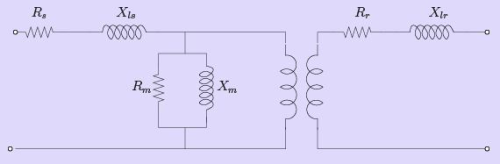Figure 16: Induction machine with the rotor open

This is just the normal transformer equivalent circuit (why? ). Measurements aregenerally made on the stator side and the rotor, in most circumstances, is shorted (if required, through some external circuitry). Since most of the electrical interaction is from the stator, it makes sense to refer all parameters to the stator.

Let us consider the rotor to be shorted. Let the steady speed attained by the rotor be ωr and the synchronous speed be ωs. The induced voltage on the rotor is now proportional to the slip i.e., slip times the induced voltage under open circuit (why? ). Further, the voltage induced and the current that ﬂows in the rotor is at a frequency equal to slip times the stator excitation frequency (why? ). The equivalent circuit can be made to represent this by shorting the secondary side and is shown in ﬁg. 17.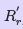and Xlrefer to the rotor resistance and leakage resistance referred to the stator side (using the square of the turns ratio, as is done in transformer). The secondary side loop is excited by a voltage sE1 , which is also at a frequency sf1 . This is the reason why the rotor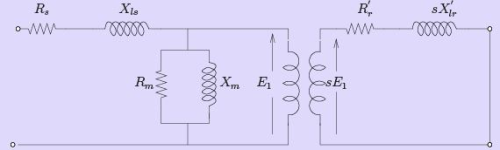Figure 17: Equivalent circuit : rotor at its own frequency

leakage is s Xl now . The current amplitude in the rotor side would therefore be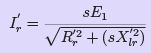(6)
This expression can be modiﬁed as follows (dividing numerator and denominator by s)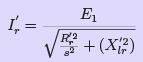(7)

Equation 7 tells us that the rotor current is the same as the current ﬂowing in a circuit with a load impedance consisting of a resistance Rr /s and inductive reactance Xl. This current would also now be at the frequency of E1 (stator frequency). Note that the slip no longer multiplies the leakage reactance. Further this current is now caused by a voltage of E1 itself (no multiplying factor of s). Hence the transformer in ﬁg. 17 can also be removed.

Since, with this, the conversion to slip frequency is no longer there, the equivalent circuit can be represented as in ﬁg. 18.

This is then the per-phase equivalent circuit of the induction machine, also called as exact equivalent circuit. Note that the voltage coming across the magnetizing branch is the applied stator voltage, reduced by the stator impedance drop. Generally the stator impedance drop is only a small fraction of the applied voltage. This fact is taken to advantage and the magnetizing branch is shifted to be directly across the input terminals and is shown in ﬁg. 19.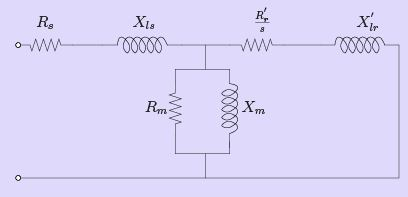Figure 18: The Exact equivalent circuit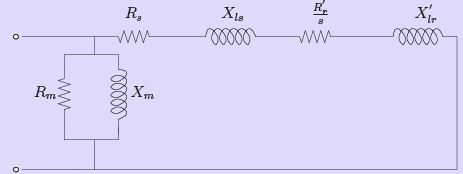Figure 19: The approximate equivalent circuit

This circuit, called the approximate equivalent circuit, is simple to use for quick calculations.
The resistance term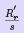could be split into two parts.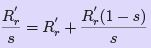(8)
With this equation the equivalent circuit can be modiﬁed as shown in ﬁg. 20.

Dividing the equation for the rotor current by s and merging the two sides of the transformer is not just a mathematical jugglery. The power dissipated in the rotor resistance (per phase) is obviously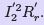From the equivalent circuit of ﬁg. 20 one can see that the rotor current (referred to stator of course) ﬂows through a resistance/s which has a component(1 − s)/s in addition to, which also dissipates power. What does this represent?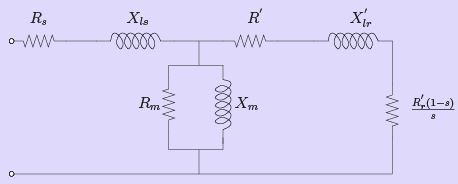Figure 20: The exact equivalent circuit - separation of rotor resistance

From the equivalent circuit, one can see that the dissipation in Rs represents the stator loss, and dissipation in Rm represents the iron loss. Therefore, the power absorption indicated by the rotor part of the circuit must represent all other means of power consumption -the actual mechanical output, friction and windage loss components and the rotor copper loss components. Since the dissipation inis rotor copper loss, the power dissipation in(1 − s)/s is the sum total of the remaining. In standard terminology, dissipation in

•/s is called the air gap power.
•is the rotor copper loss.
•(1 − s)/s is the mechanical output.

In an ideal case where there are no mechanical losses, the last term would represent the actual output available at the shaft. Out of the power Pg Transferred at the air gap, a fraction s is dissipated in the rotor and (1 − s) is delivered as output at the shaft. If there are no mechanical losses like friction and windage, this represents the power available to the load.

Offer running on EduRev: Apply code STAYHOME200 to get INR 200 off on our premium plan EduRev Infinity!

## Electrical Machines for Electrical Engg.

22 videos|41 docs|26 tests

,

,

,

,

,

,

,

,

,

,

,

,

,

,

,

,

,

,

,

,

,

;